Mobile QR Code## I. INTRODUCTION

In recent decades, the internet of things (IoT) market has been growing rapidly, and the demand for IoT sensor devices has also been increasing. Most of the currently commercialized IoT sensor devices use a 2.4 GHz ISM band. One of the most popular communication protocols is Bluetooth low energy (BLE). BLE requires relaxed specifications in terms of sensitivity or noise figure (NF), compared to cellular applications. However, BLE receivers require low-power consumption for a low replacement period of the battery and long term use. Therefore, to reduce power consumption while maintaining key performances, research works for the low-power receiver are actively being conducted (1-12).

In this paper, a 2.4-GHz BLE receiver adopting a quadrature LNA, which was recently proposed in (8), is considered and analyzed in detail. The proposed quadrature LNA is composed of a common-source (CS) amplifier with inductive degeneration and a quadrature generator based on a common-gate (CG) amplifier with a single RC network. In Section II, the proposed quadrature transconductor topology is introduced. The detailed circuit implementation of the BLE receiver is presented in Section III. The simulation results are discussed in Section IV. Finally, Section V concludes this paper.

Fig. 1. Conventional quadrature transconductor topologies (a) CS transconductor with capacitive degeneration, (b) CG transconductor with a single RC network.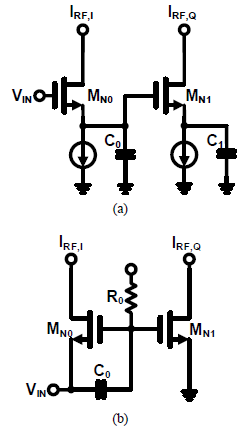## II. PROPOSED QUADRATURE TRANSCONDUCTOR TOPOLOGY

In this section, the conventional and proposed quadrature transconductor topologies are introduced. To avoid additional power consumption in the LO chain, the quadrature transconductor can be used to implement the single-quadrature low-IF receiver. Fig. 1 shows conventional quadrature transconductor topologies. In the quadrature transconductor with the CS-based topology shown in Fig. 1(a), quadrature signals are generated using the degeneration capacitors of -C and C1. When gm1 << sC1 and gm1 = ωC0, IRF,I and IRF,Q have a quadrature relationship of |IRF,IIRF,Q| = 1 and ${\angle}$(IRF,IIRF,Q) = 90 $^{\circ}$ (9,10,14). It is beneficial that the CS-based transconductor does not induce severe loading effects on the preceding block when used as an inter-stage block because of the capacitive input impedance. However, the transconductor requires input power matching to a 50-ohm terminal impedance interface when used as the first block of the receiver such as the LNA and low-noise transconductance amplifier (LNTA). In (9), the CS-based transconductor used an additional resistor for input matching. Consequently, the quadrature LNTA of (9) demonstrated poor NF performance. The CG-based quadrature transconductor topology is shown in Fig. 1(b). Low-pass and high-pass filters are implemented in the CG and CS stages, respectively, through the single RC network. The output currents of IRF,I and IRF,Q are given by

##### (1)
$$I_{R F, I}=\frac{-1}{1+s R_{0} C_{0}} g_{m 0} V_{I N}$$

##### (2)
$$I_{RF,Q}=\frac{sR_{0}C_{0}}{1+sR_{0}C_{0}}g_{m1}V_{IN}$$

where R0 and C0 are the resistance and capacitance of the RC network, respectively (11). When ωR0C0 = 1 and gm0 = gm1, the output currents of IRF,I and IRF,Q have a quadrature relationship. The CG-based quadrature transconductor has the advantage of broadband input matching when used in the first block such as LNA and LNTA. However, in terms of noise performance, the CG-based transconductor cannot achieve excellent NF.

The proposed quadrature transconductor topology is shown in Fig. 2. The proposed quadrature transconductor combines the CS amplifier with inductive degeneration and the CG-based quadrature generator with a single RC network. It takes advantage of both low NF of the CS amplifier and quadrature generation of the CG-based transconductor. It performs simultaneous noise and input power matching by employing an additional capacitor of CEX (15). The output currents of the proposed quadrature transconductor can be expressed by

##### (3)
$$I_{RF,I}=Q_{IN}g_{m0}\frac{1}{g_{m1}+sC_{0}}g_{m1}V_{IN}$$

##### (4)
$$I_{RF,Q}=- Q_{IN}g_{m0}\frac{sR_{0}C_{0}}{g_{m1}+sC_{0}}g_{m2}V_{IN}$$

where QIN is the quality factor of the input matching network when an input matching condition is met. When gm1 = gm2 and ωR0C0 = 1, IRF,I and IRF,Q have a quadrature relationship of |IRF,I IRF,Q|=1 and ${\angle}$(IRF,IIRF,Q) = 90$^{\circ}$. The proposed quadrature transconductor has lower NF performance than the previous CG-based quadrature transconductor in (11) with the same power consumption. Therefore, the proposed quadrature transconductor topology is a suitable solution for designing the quadrature LNA for low-power BLE receivers.

## III. CIRCUIT IMPLEMENTATION

In this section, the detailed circuit design of the implemented BLE receiver is presented. The block diagram of the proposed BLE receiver is shown in Fig. 3. A low-IF single-quadrature receiver topology, which consists of the quadrature LNA, a double-balanced active mixer, and the second-order Gm-C complex filter, is employed for low 1/f noise and high integration.

Fig. 5. Simulated gain and phase error of the quadrature LNA according to PVT variations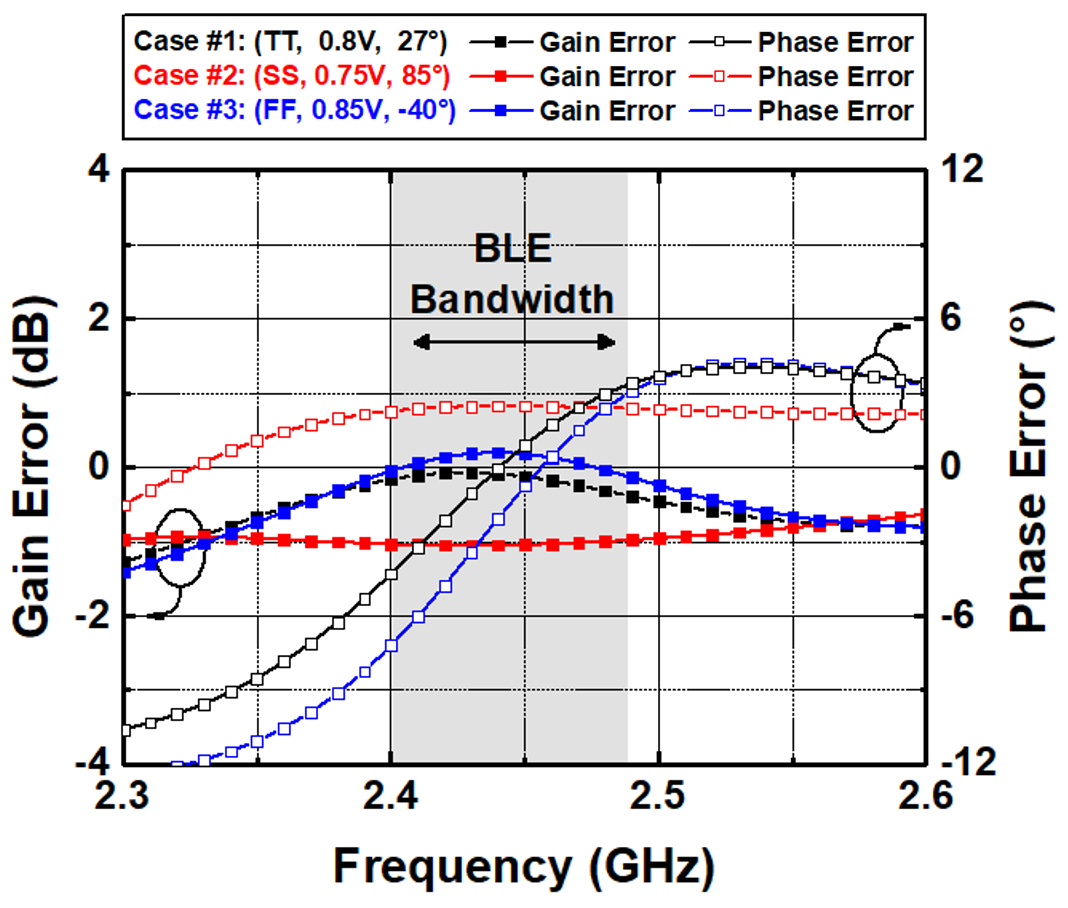The proposed quadrature LNA is shown in Fig. 4. The LNA employs the proposed quadrature transconductor to achieve low noise performance and provide quadrature output currents. It also uses an LC tank as a load. MN1 and MN2 improve reverse isolation as well as the generation of the quadrature signals. To minimize the die area, a wire bonding inductor is used to implement LS. A 4-bit-capacitor array, which is digitally controlled, is used in the LC tank to compensate for the variation in the 2.4-GHz resonant frequency of the LC tank caused by variations in the process, voltage, and temperature. The external inductor LM is used for input impedance matching. Fig. 5 shows the simulated gain and phase error of the proposed quadrature LNA in the BLE operating frequencies according to the process, voltage, and temperature (PVT) variations. The LNA can provide accurate quadrature signals in the BLE band at the typical corner case (Case #1), with gain and phase error less than 0.3 dB and 4.3$^{\circ}$, respectively. It is sufficient to meet the IRR requirement of 21 dB for the BLE standard. In addition, the gain and phase mismatch can be calibrated by tuning R0.

The noise factor of the proposed quadrature LNA can be expressed to

##### (5)
$F=1+\frac{V_{MN0}^{2}+V_{MN1}^{2}+V_{MN2}^{2}+V_{MN3}^{2}+V_{R0}^{2}}{4kTR_{S}A_{Vtot}^{2}}$,

where k is the Boltzmann’s constant, T is the absolute temperature, RS is a source resistance, AVtot is the voltage gain from the voltage source VS to the output. VMN0, VMN1, VMN2, VMN3, and VR0 represent the output-referred noise voltage generated by MN0, MN1, MN2, MN3, and R0, respectively (15). When it is assumed that ωR0C0 = 1, and ro >> ZL, A2Vtot can be approximately given to

##### (6)
$$A_{Vtot}^{2}\approx Q_{IN}^{2}\frac{\left(g_{m0}R_{0}\right)^{2}}{1+\left(g_{m1}R_{0}\right)^{2}}\left(g_{m2}Z_{L}\right)^{2}$$

where QIN is the quality factor of the input matching network and ZL is the impedance of the LC tank. The noise factor F is approximately given to

##### (7)
$$F\approx 1+\frac{\gamma }{Q_{IN}^{2}g_{m0}R_{S}}\left(1+\frac{g_{m1}}{g_{m0}}+\frac{1+g_{m1}^{2}R_{0}^{2}}{g_{m0}g_{m2}R_{0}^{2}}\right)$$ $$+\frac{1}{Q_{IN}^{2}g_{m0}R_{S}}\left(\frac{1+g_{m1}^{2}R_{0}^{2}}{g_{m0}R_{0}}\right)$$

where the second to fourth terms represent noise contributions of MN0, MN1, and MN2, respectively, and the last term represents the noise contribution of the R0. The noise contribution of MN3 is neglected because the drain node of MN3 is AC grounded. As shown in (7), MN0 determines an overall NF, and the noise contribution of MN2 and R0 are also dominant. In this design, appropriate values of gm, R0 and C0 are chosen to have an excellent NF and IRR performance through thorough layout parasitic extraction simulation.

Fig. 6. Schematic of single-to-differential double-balanced active mixer.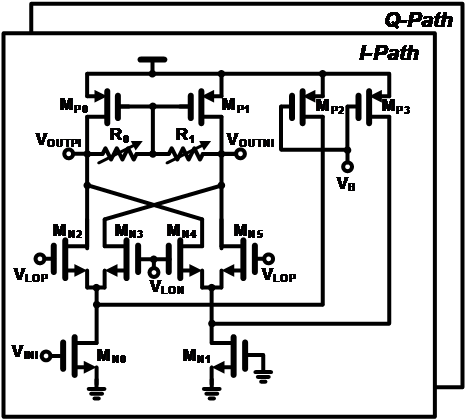Fig. 7. Schematic of the second-order Gm-C complex filter.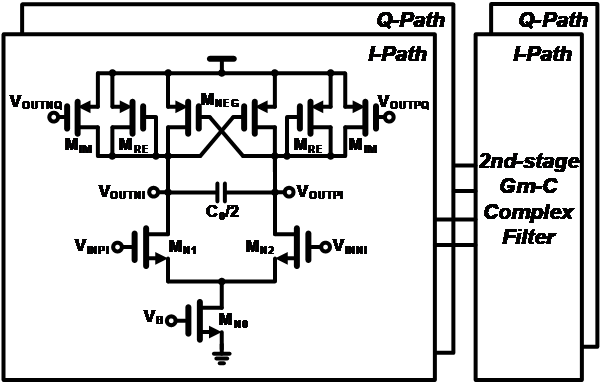### 2. Double-balanced Active Mixer

Fig. 6 shows the implemented active mixer, which is designed based on a gilbert cell. One of the differential inputs is connected to an AC ground to perform a single to differential conversion. The current bleeding technique is used to reduce the contribution of the 1/f noise. Because the quadrature signals are generated in the quadrature LNA of the RF signal path, the switching stage of the active mixer is driven by in-phase differential signals, rather than in-phase and quadrature-phase differential signals.

Table 1. Power breakdown of the implemented BLE receiver

 Block Current consumption Power consumption Quadrature LNA 1 mA 0.8 mW Active mixer 0.9 mA 0.72 mW Complex filter 0.3 mA 0.24 mW Total 2.2 mA 1.76 mW

### 3. Second-order Gm-C Complex Bandpass Filter

The second-order Gm-C complex bandpass filter, which is introduced in (16), is employed in the implemented BLE receiver and is shown in Fig. 7. It simultaneously performs image rejection and channel selection without having a loading effect on the preceding circuit. The Gm-C filter topology is advantageous in terms of power consumption. The filter has a real-pole (ωRE) and an imaginary pole (ωIM), which are expressed by (gm,RE - gm,NEG)/C0and gm,IM/C0,respectively. The real-pole determines the 3-dB bandwidth of the filter, and imaginary-pole shifts the real poles to the complex location in ωRE-jωIM,. Consequently, the filter has a bandpass frequency response. A two-stage second-order Gm-C filter is designed to satisfy the 21 dB IRR requirement for the BLE standard.

## IV. SIMULATION RESULTS

The proposed low-power receiver adopting a quadrature LNA for BLE applications was designed in a 65-nm CMOS process. The layout of the BLE receiver is depicted in Fig. 8. The active area without the bond pads is 2.24 mm$^{2}$. It draws a DC bias current of 2.2 mA from a supply voltage of 0.8 V. The power breakdown of the designed BLE receiver is shown in Table 1. The current consumptions of the LNA, mixer, and complex filter are 1 mA, 0.9 mA, and 0.3 mA, respectively.

Table 2. Simulated performance summaries of the proposed BLE receiver and comparison with previous state-of-the-art works

 Unit TMTT2017 (2) JSSC2018 (3) TMTT2019 (5) JSSC2010 (10) JSSC2015 (11) MWCL2019 (12) This Work Application - BLE BLE BLE Zigbee BLE IoT BLE Process - 28 nm CMOS 28 nm CMOS 130 nm CMOS 90 nm CMOS 130 nm CMOS 65 nm CMOS 65 nm CMOS Architecture - Low-IF Low-IF Low-IF Low-IF Low-IF Low-IF Low-IF Frequency GHz 2.4-2.48 2.4-2.48 2.4-2.48 2.4-2.48 2.4-2.48 0.9-0.92 2.4-2.48 Gain dB 46 41.3 42 76 56.1 40.7 53.2 NF dB 6.5 8.8 7.2 10 15.1 1.94 4.81 IRR dB 26 25.1 40 35 30.5 NR2) 38 Pdc mW 2.751) 1.3 1.71) 3.6 0.6 3.6 1.76 Supply Voltage V 1 0.3 1.2 1.2 0.8 1.8 0.8 Area mm2 1.92 1.65 0.7 0.23 0.25 0.559 2.24

1) It includes power consumption of the demodulator.

2) NR: Not Reported

Fig. 12. Simulated conversion gain, IRR, and NF versus RF frequencies.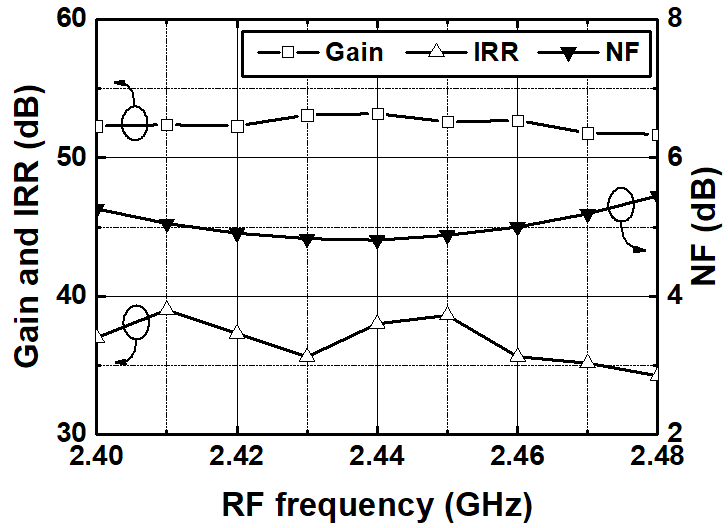Fig. 9 shows the input return loss (S11) of the receiver. The simulated S11 is lower than -8 dB over the BLE band from 2.4 - 2.48 GHz. The simulated NF of the receiver in the 2 MHz IF frequency is shown in Fig. 10. The designed BLE receiver has a minimum NF of 4.81~dB in 2.44 GHz RF frequency. Fig. 11 shows the frequency response of the receiver. The simulated maximum gain is 53.2 dB in an IF frequency of 2 MHz. The simulated maximum IRR over the BLE operating frequencies is 38 dB. Fig. 12 shows the maximum conversion gain, IRR, and NF versus RF frequencies. The maximum conversion gain and IRR are obtained as 51.7 to 53.2 dB, and 34.2 to 39 dB, respectively. The receiver achieves an NF performance of less than 5.45 dB in all the BLE operating frequencies. Fig. 13 shows the simulated IRR performance according to PVT variations at 2.44 GHz RF frequency. The obtained IRRs are more than 33 dB for all corner cases. These are sufficient to meet the IRR requirement of 21 dB. Table 2 summarizes and compares the performance of the proposed receiver with previous state-of-the-art works. As shown in the Table 2, the proposed receiver demonstrates lower NF performance compared to previous works using the quadrature LNA and LNTA of (10,11).

## V. CONCLUSIONS

### ACKNOWLEDGMENTS

This work was supported in part by the Basic Science Research Program through the National Research Foundation of Korea (NRF) funded by the Ministry of Education under Grant NRF-2018R1D1A1B07042804 and in part by the Ministry of Science and ICT, Korea, under the Information Technology Research Center Support Program supervised by the Institute for Information and Communications Technology Promotion (IITP) under Grant IITP-2020-2018-0-01433. The chip fabrication and EDA tool were supported by the IC Design Education Center (IDEC), Korea.

### REFERENCES

1
Balankutty A., March 2010, A 0.6-V zero-IF/low-IF receiver with integrated fractional-N synthesizer for 2.4-GHz ISM-band applications, IEEE J. Solid-State Circuits, Vol. 45, No. 3, pp. 538-5532
Binsfeld Ferreira S., May 2017, System Design of a 2.75-mW Discrete-Time Superheterodyne Receiver for Bluetooth Low Energy, IEEE Trans. Microw. Theory Tech., Vol. 65, No. 5, pp. 1904-19133
Yi H., June 2018, A 0.18-V 382 μW Bluetooth low-energy receiver front-end with 1.33-nW sleep power for energy-harvesting applications in 28-nm CMOS, IEEE J. Solid-State Circuits, Vol. 53, No. 6, pp. 1618-16274
Lee S., May 2018, Ultralow-power 2.4-GHz receiver with all passive sliding-IF mixer, IEEE Trans. Microw. Theory Tech, Vol. 66, No. 5, pp. 2356-23625
Silva-Pereira M., Jan 2019, A 1.7-mW −92-dBm sensitivity low-IF receiver in 0.13-μm CMOS for Bluetooth LE applications, IEEE Trans. Microw. Theory Tech., Vol. 67, No. 1, pp. 332-3466
Thijssen B. J., Feb. 2020, A 370 µW 5.5dB-NF BLE/BT5.0/IEEE 802.15.4-compliant receiver with > 63dB adjacent channel rejection at >2 channels offset in 22nm FDSOI, in IEEE Int. Solid-State Circuits Conf. Tech. Dig., San Francisco, CA, pp. 466-4687
Tamura M., Feb 2020, A 0.5V BLE transceiver with a 1.9 mW RX achieving −96.4 dBm sensitivity and 4.1 dB adjacent channel rejection at 1 MHz offset in 22 nm FDSOI, in IEEE Int. Solid-State Circuits Conf. Tech. Dig., San Francisco, CA, pp. 468-4708
Park B., Feb 2020, BLE receiver employing new quadrature LNA for IoT application, the 27th Korean Conference on Semiconductors9
Liscidini A., Feb 2008, A 2.4 GHz 3.6 mW 0.35 mm2 quadrature front-end RX for ZigBee and WPAN applications, IEEE Int. Solid-State Circuits Conf. Tech. Dig., San Francisco, CA, pp. 370-62010
Tedeschi M., Sep 2010, Low-power quadrature receivers for ZigBee (IEEE 802.15.4) applications, IEEE J. Solid-State Circuits, Vol. 45, No. 9, pp. 1710-171911
Selvakumar A., Dec 2015, Sub-mW current re-use receiver front-end for wireless sensor network applications, IEEE J. of Solid-State Circuits, Vol. 50, No. 12, pp. 2965-297412
Kim S., Sept 2019, A low-power RF-to-BB-current-reuse receiver employing simultaneous noise and input matching and 1/f noise reduction for IoT applications, IEEE Microw. Wireless Compon. Lett., Vol. 29, No. 9, pp. 614-61613
 Bluetooth Specification , Dec. 2016, (Low Energy Controller); Part A, Bluetooth SIG, Vol. 5.0, No. 614
Kwon K., Nov 2010, A 5.8 GHz integrated CMOS dedicated short range communication transceiver for the Korea/Japan electronic toll collection system, IEEE Trans. Microw. Theory Tech., Vol. 58, No. 11, pp. 2751-276315
Razavi B., 2012, RF Microelectronics, 2nd ed. New York, NY, USA:Prentice-Hall16
Selvakumar A., April 2015, Current-Recycling Complex Filter for Bluetooth-Low-Energy Applications, IEEE Trans. Circuits Syst.II: Express Briefs, Vol. 62, No. 4, pp. 332-336## Author

Beomyu Park is currently working toward the integrated B.S. and M.S. degree in Department of Electronics Engineering, Kangwon National University, Chuncheon, Korea.

His research interests include CMOS RF/analog integrated circuits and RF system design for wireless communications.

Kuduck Kwon received the B.S. and Ph.D. degrees in Electrical Engi-neering and Computer Science from Korea Advanced Institute of Science and Technology (KAIST), in Dae-jeon, Korea, in 2004 and 2009, respectively.

His doctoral research concerned digital TV tuners and dedicated short-range communication (DSRC) systems.

From 2009 to 2010, he was a Post-Doctoral Researcher with KAIST, where he studied a surface acoustic wave (SAW)-less receiver and developed RF transceivers for DSRC applications.

From 2010 to 2014, he was a Senior Engineer with Samsung Electronics Co. LTD., Suwon, Korea, where he was involved in studying software-defined receiver and developing silicon tuner and cellular RFICs.

In 2014, he joined the Department of Electronics Engineering, Kangwon National University, Chuncheon, Korea, where he is currently an Associate Professor.

His research interests include CMOS mmWave/RF/analog integrated circuits and RF system design for wireless communi-cations.